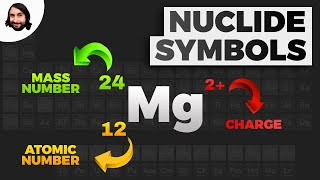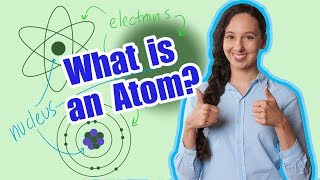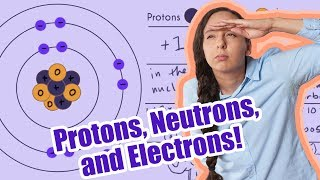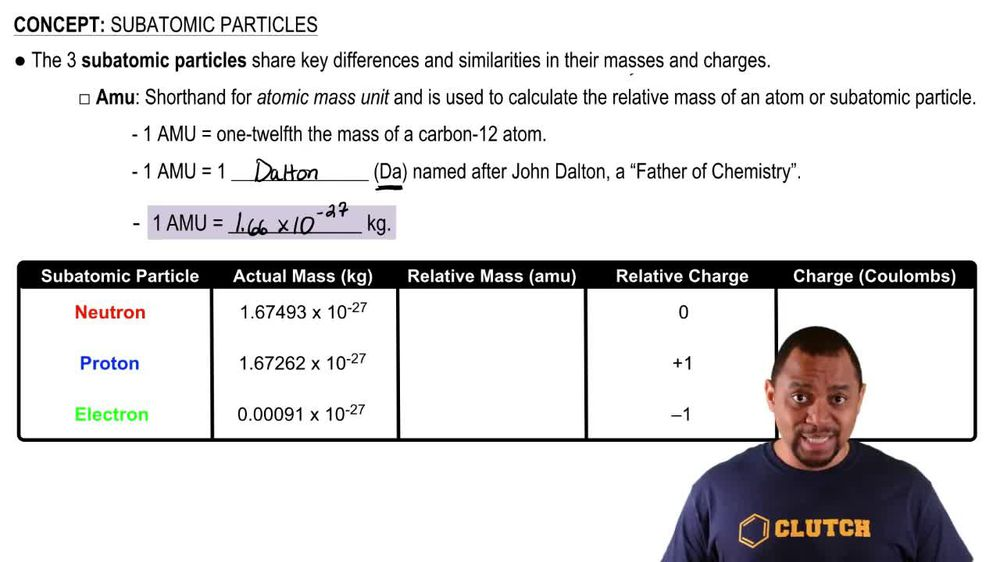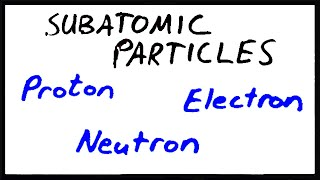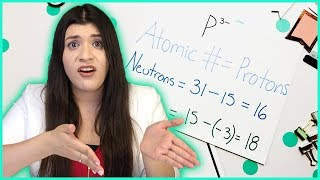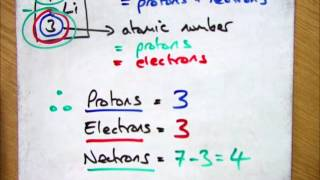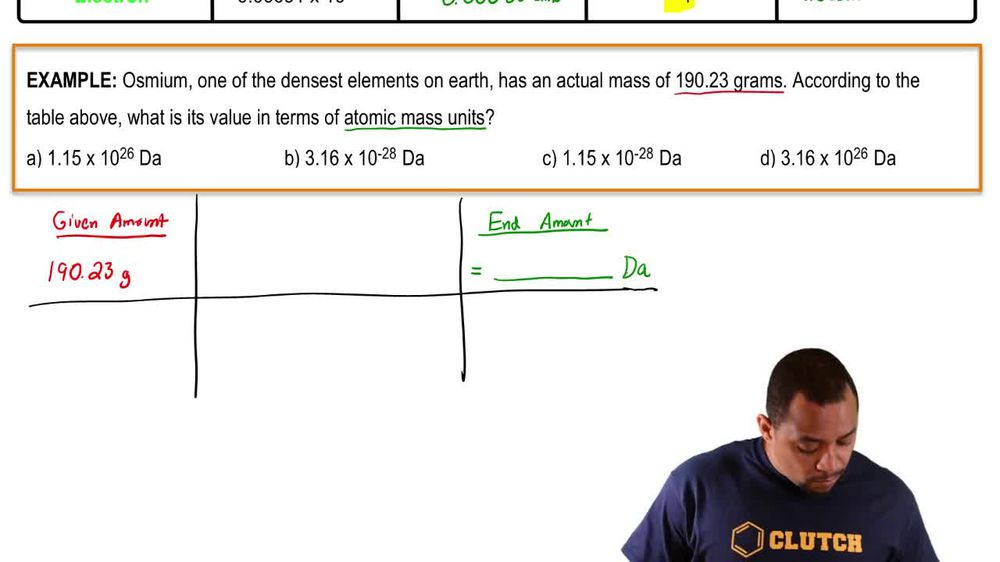Start typing, then use the up and down arrows to select an option from the list.
1. 2. Atoms & Elements2. Subatomic Particles
Problem

# How many protons, neutrons, and electrons are in the following atoms? (e) 239U

Relevant Solution4m
Play a video:
Hey everyone in this example, we need to identify the number of subatomic particles in 57 cobalt. So we should recognize that this is an isotope. We know that this is an isotope due to the fact that were given a symbol with a mass number of cobalt. So this is an isotope of cobalt and this isotope is cobalt 57. The next step is to recognize that in the prompt subatomic particles we should recognize would be our number of electrons, number of protons and our number of neutrons because these subatomic particles all characterize. And adam Now looking at our isotope symbol for Cobalt Cobalt 57, we would recognize that this 57 here, which identifies our isotope is also considered our mass number. And we should recall that the mass number is found. We can say it's equal to taking our number of protons and adding that and we'll just make this blue. Actually we can take our number of protons and add it to our number of neutrons. So right now we can figure out what those values are. But in order to do so we need to figure out our number of protons first. So to give a full symbol for a isotope or an atom in general, we also want to be sure to include the atomic number, recall that atomic number is also represented by the symbol Z. And it's typically written in the left hand subscript next to our isotope or next to the symbol or rather than adam representing our isotope, which in this case is cobalt here. So we want to go ahead and find cobalt on our periodic table. We would see that cobalt is in the transition metal D block of our periodic table In Group eight B. And we would see that it has the atomic number being 27. So that was that is what we would use to fill in for our atomic number here for our isotope symbol. And we should recall that our atomic number is going to also be equal to our number of protons. And we'll make that blue. We can say that for neutral atoms only atomic number Z is also equal to our number of electrons. And so because we found that cobalt on the periodic table has an atomic number equal to 27, we can say therefore not only do we have protons, but we also have 27 electrons. And that is due to the fact that we are given a neutral atom because we don't have a charge in our symbol for cobalt. So right now we've already found part of our answer. We have two subatomic particles identified. We have 27 protons, 27 electrons. And now we need to find our number of neutrons. So we would say that our number of neutrons can be found by taking our given mass number here, Which according to the prompt is 57, which is given in our symbol. So we can take 57 And we can subtract that from our number of protons, which we've identified as 27 protons. And this difference gives us our number of neutrons equal to a value of 30. And so we can say we have 30 neutrons. So for our final answers we have 30 neutrons. We have 27 protons and 27 electrons as we've outlined over here. So everything boxed in blue represents our final answers for our number of subatomic particles for an isotope of 57 cobalt. If you have any questions, leave them down below and I'll see everyone in the next practice video.